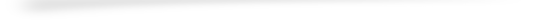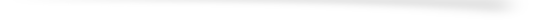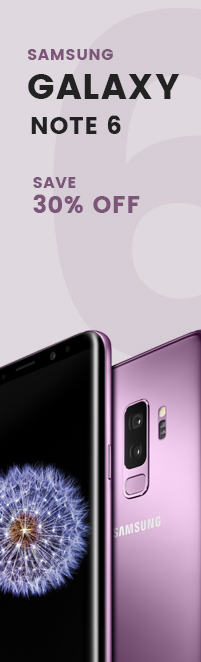L O A D I N G# latest fashion

#### save up to 50% off# latest fashion

#### save up to 50% off# latest fashion

#### save up to 50% off• xs
• s
• m
• l
• xl
• xs
• s
• m
• l
• xl
• xs
• s
• m
• l
• xl
• xs
• s
• m
• l
• xl
• xs
• s
• m
• l
• xl
• xs
• s
• m
• l
• xl
• xs
• s
• m
• l
• xl
• xs
• s
• m
• l
• xl
• xs
• s
• m
• l
• xl
• xs
• s
• m
• l
• xl
• xs
• s
• m
• l
• xl
##### size
• xs
• s
• m
• l
• xl• xs
• s
• m
• l
• xl
• xs
• s
• m
• l
• xl
• xs
• s
• m
• l
• xl
• xs
• s
• m
• l
• xl
• xs
• s
• m
• l
• xl
• xs
• s
• m
• l
• xl
• xs
• s
• m
• l
• xl
• xs
• s
• m
• l
• xl
• xs
• s
• m
• l
• xl
• xs
• s
• m
• l
• xl
• xs
• s
• m
• l
• xl
##### size
• xs
• s
• m
• l
• xl• xs
• s
• m
• l
• xl
• xs
• s
• m
• l
• xl
• xs
• s
• m
• l
• xl
• xs
• s
• m
• l
• xl
• xs
• s
• m
• l
• xl
• xs
• s
• m
• l
• xl
• xs
• s
• m
• l
• xl
• xs
• s
• m
• l
• xl
• xs
• s
• m
• l
• xl
• xs
• s
• m
• l
• xl
• xs
• s
• m
• l
• xl
##### size
• xs
• s
• m
• l
• xl• xs
• s
• m
• l
• xl
• xs
• s
• m
• l
• xl
• xs
• s
• m
• l
• xl
• xs
• s
• m
• l
• xl
• xs
• s
• m
• l
• xl
• xs
• s
• m
• l
• xl
• xs
• s
• m
• l
• xl
• xs
• s
• m
• l
• xl
• xs
• s
• m
• l
• xl
• xs
• s
• m
• l
• xl
• xs
• s
• m
• l
• xl
##### size
• xs
• s
• m
• l
• xl• xs
• s
• m
• l
• xl
• xs
• s
• m
• l
• xl
• xs
• s
• m
• l
• xl
• xs
• s
• m
• l
• xl
• xs
• s
• m
• l
• xl
• xs
• s
• m
• l
• xl
• xs
• s
• m
• l
• xl
• xs
• s
• m
• l
• xl
• xs
• s
• m
• l
• xl
• xs
• s
• m
• l
• xl
• xs
• s
• m
• l
• xl
• xs
• s
• m
• l
• xl

• xs
• s
• m
• l
• xl
• xs
• s
• m
• l
• xl
• xs
• s
• m
• l
• xl
• xs
• s
• m
• l
• xl
• xs
• s
• m
• l
• xl
• xs
• s
• m
• l
• xl
• xs
• s
• m
• l
• xl
• xs
• s
• m
• l
• xl
• xs
• s
• m
• l
• xl
• xs
• s
• m
• l
• xl
• xs
• s
• m
• l
• xl
• xs
• s
• m
• l
• xl
• xs
• s
• m
• l
• xl
• xs
• s
• m
• l
• xl
• xs
• s
• m
• l
• xl
• xs
• s
• m
• l
• xl
• xs
• s
• m
• l
• xl
• xs
• s
• m
• l
• xl
• xs
• s
• m
• l
• xl
• xs
• s
• m
• l
• xl
• xs
• s
• m
• l
• xl
• xs
• s
• m
• l
• xl
• xs
• s
• m
• l
• xl
• xs
• s
• m
• l
• xl
• xs
• s
• m
• l
• xl
• xs
• s
• m
• l
• xl
• xs
• s
• m
• l
• xl
• xs
• s
• m
• l
• xl
• xs
• s
• m
• l
• xl
• xs
• s
• m
• l
• xl
• xs
• s
• m
• l
• xl
• xs
• s
• m
• l
• xl
• xs
• s
• m
• l
• xl
• xs
• s
• m
• l
• xl
• xs
• s
• m
• l
• xl
• xs
• s
• m
• l
• xl
• xs
• s
• m
• l
• xl
• xs
• s
• m
• l
• xl
• xs
• s
• m
• l
• xl
• xs
• s
• m
• l
• xl
• xs
• s
• m
• l
• xl
• xs
• s
• m
• l
• xl
• xs
• s
• m
• l
• xl
• xs
• s
• m
• l
• xl
• xs
• s
• m
• l
• xl
• xs
• s
• m
• l
• xl
• xs
• s
• m
• l
• xl
• xs
• s
• m
• l
• xl
• xs
• s
• m
• l
• xl
• xs
• s
• m
• l
• xl
• xs
• s
• m
• l
• xl
• xs
• s
• m
• l
• xl
• xs
• s
• m
• l
• xl
• xs
• s
• m
• l
• xl
• xs
• s
• m
• l
• xl
• xs
• s
• m
• l
• xl
• xs
• s
• m
• l
• xl
• xs
• s
• m
• l
• xl
• xs
• s
• m
• l
• xl
• xs
• s
• m
• l
• xl
• xs
• s
• m
• l
• xl
• xs
• s
• m
• l
• xl
• xs
• s
• m
• l
• xl
• xs
• s
• m
• l
• xl
• xs
• s
• m
• l
• xl
• xs
• s
• m
• l
• xl
• xs
• s
• m
• l
• xl
• xs
• s
• m
• l
• xl
• xs
• s
• m
• l
• xl
• xs
• s
• m
• l
• xl
• xs
• s
• m
• l
• xl
• xs
• s
• m
• l
• xl
• xs
• s
• m
• l
• xl
• xs
• s
• m
• l
• xl
• xs
• s
• m
• l
• xl
• xs
• s
• m
• l
• xl
• xs
• s
• m
• l
• xl
• xs
• s
• m
• l
• xl
• xs
• s
• m
• l
• xl
##### size
• xs
• s
• m
• l
• xl## success story##### 25 july 2018

Sometimes on purpose ected humour. dummy text.##### 25 july 2018

Sometimes on purpose ected humour. dummy text.##### 25 july 2018

Sometimes on purpose ected humour. dummy text.##### 25 july 2018

Sometimes on purpose ected humour. dummy text.##### 25 july 2018

Sometimes on purpose ected humour. dummy text.##### 25 july 2018

Sometimes on purpose ected humour. dummy text.

## customer says###### mark jecno

Long established fact that a reader will be distracted by the readable.Long established fact.###### lara oprt

Many desktop publishing packages and web page editors now use Lorem Ipsum as their default model text, and a search for text.###### mark jecno

Long established fact that a reader will be distracted by the readable.Long established fact.###### lara oprt

Many desktop publishing packages and web page editors now use Lorem Ipsum as their default model text, and a search for text.